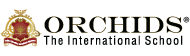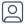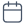Swipe UpI hope you enjoy reading this blog post.
Call Us to know more about Orchids

# Consecutive NumbersPrakriti Dhodare

.2023-09-05

.

## Introduction

Kids use math skills daily, whether at school or home, during their routines or play. Learning mathematics is essential for kids from an early age. You can foster a positive attitude towards mathematics in your kids by making it easy for them. Mathematics is not something you can just read and learn. There are various formulas and derivations in mathematics. To make mathematics easy for your kids, you need to make them understand each concept of mathematics. Let’s start with what is a consecutive number and learn more about consecutive numbers with examples.

## What are Consecutive Numbers?

The numbers that follow each other continuously, from increasing to decreasing order, are called consecutive numbers. Numbers are always written in a series where they must not skip any in between, and the difference between them is fixed. Usually, there is a difference of 1 between every two consecutive numbers. Now you know what are consecutive numbers. Let’s see a few consecutive number examples.

## Consecutive Number Example:

### What are the 7 consecutive numbers?

1, 2, 3, 4, 5, 6, and 7 are the 7 consecutive numbers. It is not mandatory to start it at 1. The concept of seven consecutive numbers can be applied to 55, 56, 57, 58, 59, 60, 61, and 62 as well. Below are some more examples of seven consecutive numbers.

Such as :

• 11, 12, 13, 14, 15, 16, 17
• -3, -2, -1, 0, 1, 2, 3
• 45, 46, 47, 48, 49, 50, 51
• 37, 38, 39, 40, 41, 42, 43
Consecutive multiples of 5

Consecutive Odd numbers between 11 to 21

Consecutive multiples of 3 upto 18
5,10,15, 20, 25
11,13,15,17,19, 21
3, 6, 9,12,15,18
3, 6, 9,12,15,18, 21, 24
7,9,11,13,15,17,19

## What is the HCF of Two Consecutive Numbers?

The HCF of two consecutive numbers is always 1. This is because the two consecutive numbers do not have any common factor other than 1. Hence, 1 proves to be the only HCF between two consecutive numbers.

## What Are Consecutive Composite Numbers?

Consecutive Composite Numbers are made up of more than two factors, and the list of the numbers without skipping any number in between is called a consecutive composite number. All natural numbers that are not prime numbers, except 1, are called composite numbers.

### Examples of consecutive composite numbers.

• 4, 6, 8, 9, 10
• 95, 96, 98, 99, 100

## What Are Consecutive Natural Numbers?

A subset of real numbers that only includes positive integers like 1, 2, 3, 4, 5, 6, and so on is called a ” natural number. The numbers that continuously follow each other in order from the smallest number to the largest number are called consecutive natural numbers. It excludes zero, fractions, decimals, and negative numbers.

### Examples of consecutive natural numbers

1,2,3,4,5,6,7,8,9 upto infinity

Here, zero is not a natural number because 0 is neither positive nor negative.

## What Are Consecutive Odd Numbers?

Odd consecutive numbers are those whole numbers that can be divided by 2, leaving the remainder as 1. The list flow of even numbers without interruption is called even consecutive numbers.

### Examples of consecutive odd numbers

• 1, 3, 5, 7, 9, 11
• 53, 55, 57, 59, 61

## What Are Consecutive Even Numbers?

Even consecutive numbers are whole numbers that cannot be divided entirely by 2. The list flow of even numbers without interruption is called even consecutive numbers.

### Examples of consecutive even numbers

• 0, 2, 4, 6, 8, 10
• 28, 30, 32, 34, 36

## What Are Consecutive Prime Numbers?

Numbers with exactly 2 factors, 1 and the number itself, are called prime numbers. The series of prime numbers is said to be consecutive prime numbers.

### Examples of consecutive prime numbers

• 2, 3, 5, 7, 11, 13, 17
• 73, 79, 83, 89, 97

## How to Find Consecutive Numbers?

Suppose, n is an integer.

Therefore, (n + 1) and (n + 2) will be the next two consecutive integers.

### 1. What Are Two Consecutive Even Numbers?

Answer: Any even number that follows another number in a sequence is said to be two consecutive even numbers.

The two consecutive even numbers are: 2, 4 or 8, 10

### 2. What Are Two Consecutive Odd Numbers?

Answer: Any odd number that follows another number in a sequence is said to be two consecutive odd numbers.

The two consecutive odd numbers are: 7, 9 or 13, 15

### 3. Find the Average of the First 6 Consecutive Even Numbers.

Answer: First n even number sum will be:

=n(n+1)

Average

=n(n+1)/n

=n+1

Suppose, the first six consecutive odd numbers are 2, 4, 6, 8, 10, 12

therefore, n = 6

=n(n+1)

=6(6+1)

=42

Sum of first six consecutive numbers is 42

Average,

= n+1

= (6+1)

= 7

### 4. Find the Average of the First 7 Consecutive Even Numbers.

Answer: First n even number sum will be:

= n (n+1)

Average

= n (n+1) / n

= n+1

Suppose, the first six consecutive odd numbers are 2, 4, 6, 8, 10, 12, 14

therefore, n = 7

= n (n+1)

= 7 (7+1)

= 56

Sum of first six consecutive numbers is 56

Average,

= n+1

= (7+1)

= 8

### 5. What Is the Formula of Consecutive Odd Numbers?

Sum of n odd numbers = n2

Derivation of Sum of odd numbers

Odd number = 1, 3, 5 ……..(2n-1)

Here, first number (a) = 1

Last number (l) = (2n-1)

Sum of AP (Arithematic Progression) = n/2 (a+l)

= n/2 (1 + 2n -1)

= 2n2 / 2

= n2

Composite Numbers

Cardinal Numbers

Inch to Centimeter Conversion: Examples with Formula

Share